Next: The Coherence Length (Pippard's Equation) Up: Magnetic Properties of Conventional Superconductors Previous: Type-I Superconductors

## The London Penetration Depth

In 1935, F. and H. London modified an essential equation of electrodynamics (i.e. Ohm's Law) in such a way as to obtain the Meissner effect, without altering Maxwell's equations themselves. In doing so, they incorporated the two-fluid model of Gorter and Casimir . The two-fluid model separates the electron system into a superconducting component with an electron density ns, and a normal component with an electron density nn. They assumed the total electron densitybehaved such that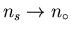as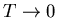, and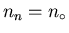when T > Tc. Emerging from their phenomenological model is the so-called London penetration depth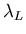: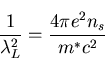(1)

where m* is the effective mass of the superconducting carriers, and ns is the superconductor carrier density. Pure-elemental metals and low concentration alloys (eg. dilute SnIn alloy system ), where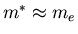(free electron mass), tend to be type-I. Generally speaking,is short and the Fermi velocity of the superconducting carriers vF is high in these materials .

The physical significance ofpertains to the failure of the Meissner effect to occur abruptly at the surface of a superconductor; rather the magnetic field penetrates slightly into the bulk of the superconducting material on a length scale given by. Consider a semi-infinite superconductor with the boundary between normal and superconducting regions at x=0. In the presence of an external magnetic field applied perpendicular to the surface, London theory predicts that the magnetic flux decays exponentially into the bulk of the superconductor according to [13,16]: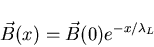(2)

where(0) is the magnetic field at the surface of the superconductor, and x is the distance into the superconductor from the surface (see Fig. 2.2). The expulsion of the magnetic field is accomplished by shielding currents (or supercurrents) which flow at the surface of the superconductor without any Ohmic losses. These supercurrents form a finite sheath which is spread out to a thicknessinto the sample. Such a distribution of current is much more energetically favourable than an infinitely thin sheath of current . The supercurrent density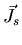is related to the local magnetic flux densityby Ampére's Law: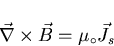(3)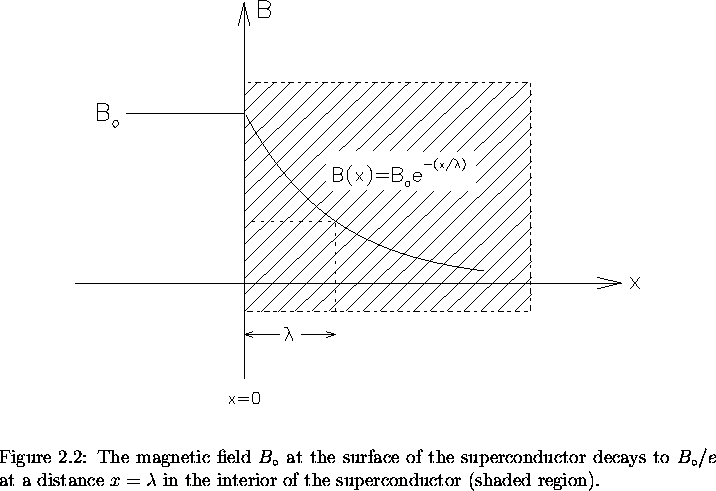Next: The Coherence Length (Pippard's Equation) Up: Magnetic Properties of Conventional Superconductors Previous: Type-I Superconductors
Jess H. Brewer
2001-09-28